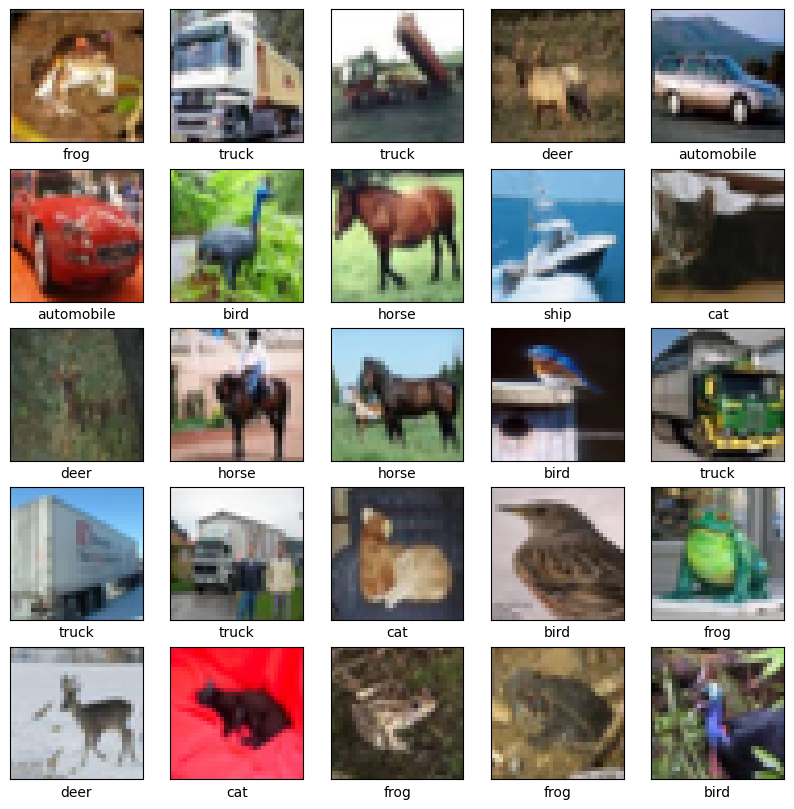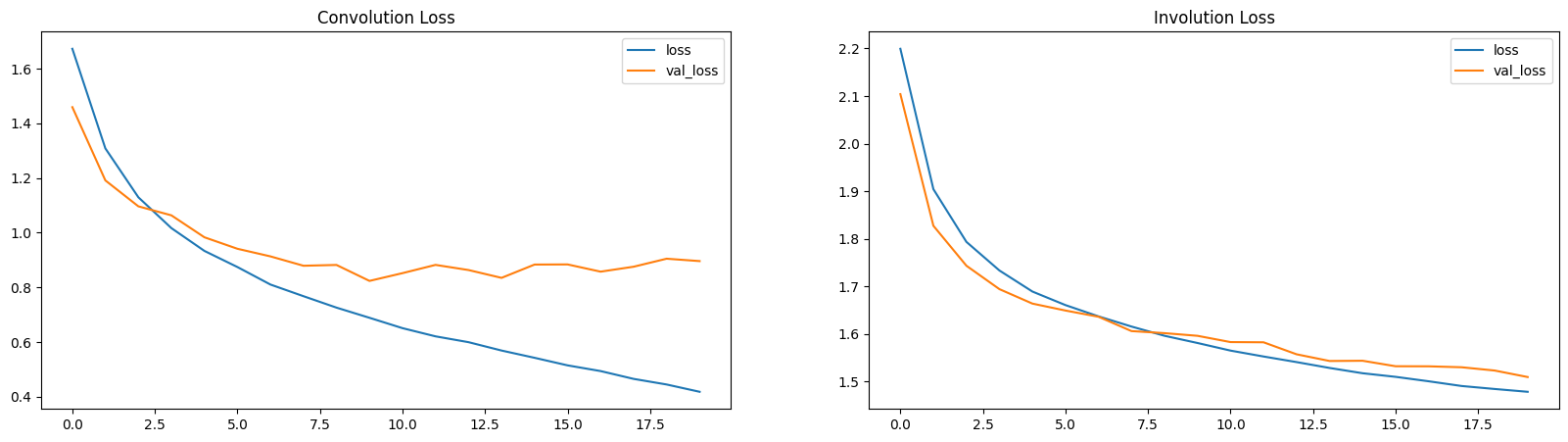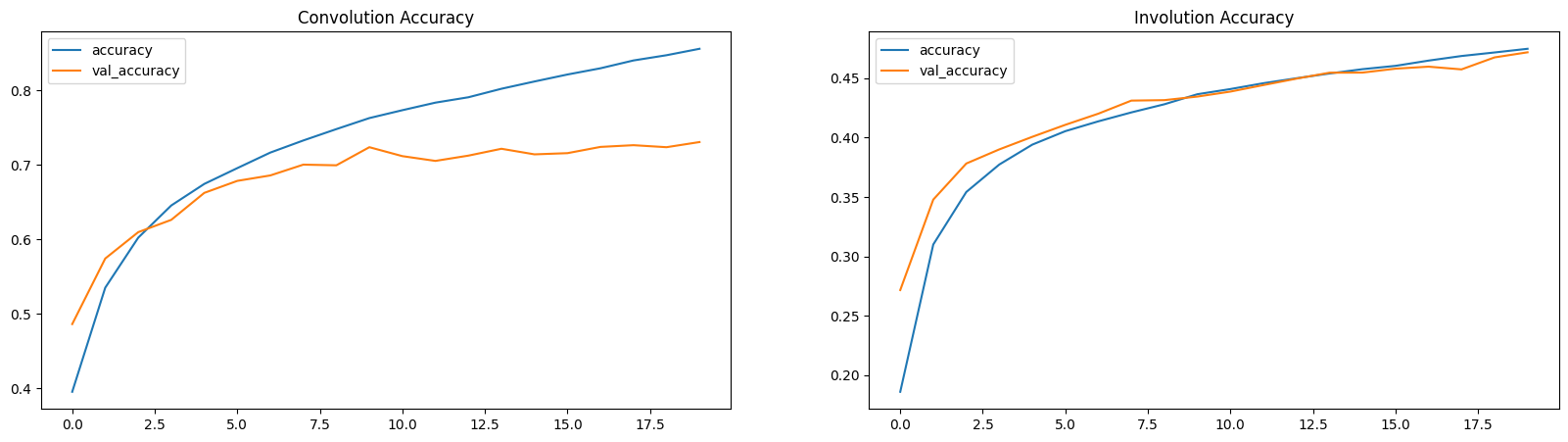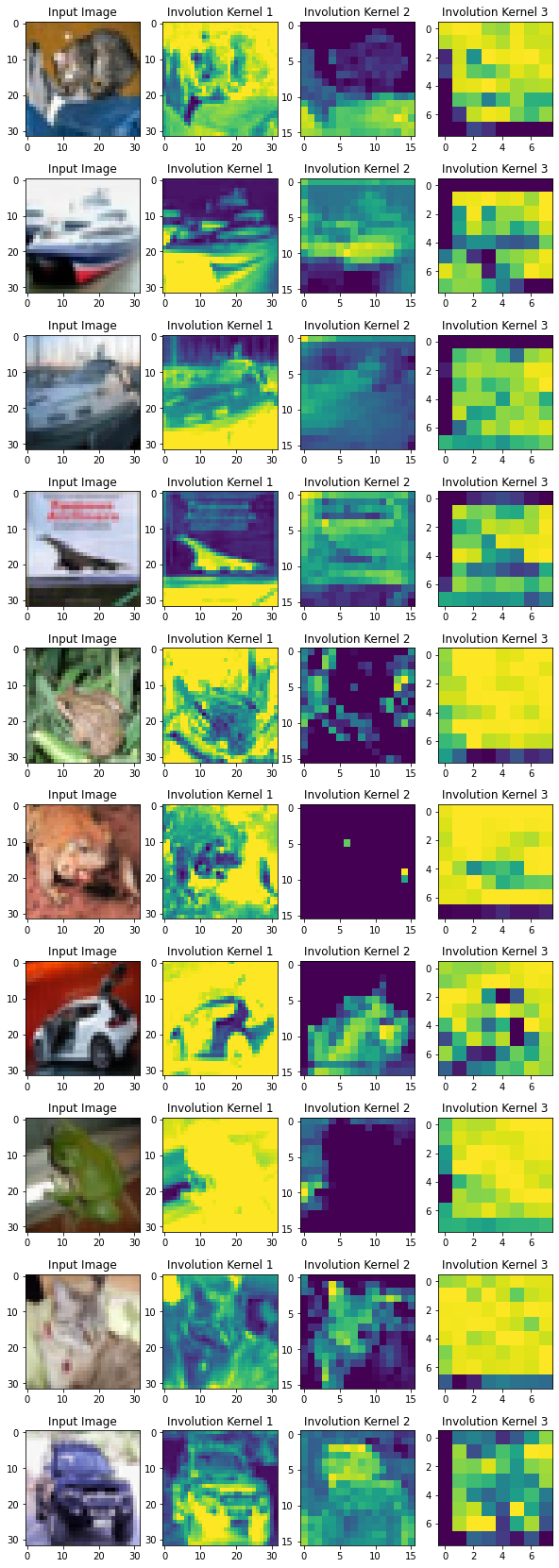» Code examples / Computer Vision / Involutional neural networks

# Involutional neural networks

Author: Aritra Roy Gosthipaty
Date created: 2021/07/25View in ColabGitHub source

Description: Deep dive into location-specific and channel-agnostic "involution" kernels.

## Introduction

Convolution has been the basis of most modern neural networks for computer vision. A convolution kernel is spatial-agnostic and channel-specific. Because of this, it isn't able to adapt to different visual patterns with respect to different spatial locations. Along with location-related problems, the receptive field of convolution creates challenges with regard to capturing long-range spatial interactions.

To address the above issues, Li et. al. rethink the properties of convolution in Involution: Inverting the Inherence of Convolution for VisualRecognition. The authors propose the "involution kernel", that is location-specific and channel-agnostic. Due to the location-specific nature of the operation, the authors say that self-attention falls under the design paradigm of involution.

This example describes the involution kernel, compares two image classification models, one with convolution and the other with involution, and also tries drawing a parallel with the self-attention layer.

## Setup

``````import tensorflow as tf
from tensorflow import keras
import matplotlib.pyplot as plt

# Set seed for reproducibility.
tf.random.set_seed(42)
``````

## Convolution

Convolution remains the mainstay of deep neural networks for computer vision. To understand Involution, it is necessary to talk about the convolution operation.Consider an input tensor X with dimensions H, W and C_in. We take a collection of C_out convolution kernels each of shape K, K, C_in. With the multiply-add operation between the input tensor and the kernels we obtain an output tensor Y with dimensions H, W, C_out.

In the diagram above `C_out=3`. This makes the output tensor of shape H, W and 3. One can notice that the convoltuion kernel does not depend on the spatial position of the input tensor which makes it location-agnostic. On the other hand, each channel in the output tensor is based on a specific convolution filter which makes is channel-specific.

## Involution

The idea is to have an operation that is both location-specific and channel-agnostic. Trying to implement these specific properties poses a challenge. With a fixed number of involution kernels (for each spatial position) we will not be able to process variable-resolution input tensors.

To solve this problem, the authors have considered generating each kernel conditioned on specific spatial positions. With this method, we should be able to process variable-resolution input tensors with ease. The diagram below provides an intuition on this kernel generation method.``````class Involution(keras.layers.Layer):
def __init__(
self, channel, group_number, kernel_size, stride, reduction_ratio, name
):
super().__init__(name=name)

# Initialize the parameters.
self.channel = channel
self.group_number = group_number
self.kernel_size = kernel_size
self.stride = stride
self.reduction_ratio = reduction_ratio

def build(self, input_shape):
# Get the shape of the input.
(_, height, width, num_channels) = input_shape

# Scale the height and width with respect to the strides.
height = height // self.stride
width = width // self.stride

# Define a layer that average pools the input tensor
# if stride is more than 1.
self.stride_layer = (
keras.layers.AveragePooling2D(
)
if self.stride > 1
else tf.identity
)
# Define the kernel generation layer.
self.kernel_gen = keras.Sequential(
[
keras.layers.Conv2D(
filters=self.channel // self.reduction_ratio, kernel_size=1
),
keras.layers.BatchNormalization(),
keras.layers.ReLU(),
keras.layers.Conv2D(
filters=self.kernel_size * self.kernel_size * self.group_number,
kernel_size=1,
),
]
)
# Define reshape layers
self.kernel_reshape = keras.layers.Reshape(
target_shape=(
height,
width,
self.kernel_size * self.kernel_size,
1,
self.group_number,
)
)
self.input_patches_reshape = keras.layers.Reshape(
target_shape=(
height,
width,
self.kernel_size * self.kernel_size,
num_channels // self.group_number,
self.group_number,
)
)
self.output_reshape = keras.layers.Reshape(
target_shape=(height, width, num_channels)
)

def call(self, x):
# Generate the kernel with respect to the input tensor.
# B, H, W, K*K*G
kernel_input = self.stride_layer(x)
kernel = self.kernel_gen(kernel_input)

# reshape the kerenl
# B, H, W, K*K, 1, G
kernel = self.kernel_reshape(kernel)

# Extract input patches.
# B, H, W, K*K*C
input_patches = tf.image.extract_patches(
images=x,
sizes=[1, self.kernel_size, self.kernel_size, 1],
strides=[1, self.stride, self.stride, 1],
rates=[1, 1, 1, 1],
)

# Reshape the input patches to align with later operations.
# B, H, W, K*K, C//G, G
input_patches = self.input_patches_reshape(input_patches)

# Compute the multiply-add operation of kernels and patches.
# B, H, W, K*K, C//G, G
output = tf.multiply(kernel, input_patches)
# B, H, W, C//G, G
output = tf.reduce_sum(output, axis=3)

# Reshape the output kernel.
# B, H, W, C
output = self.output_reshape(output)

# Return the output tensor and the kernel.
return output, kernel
``````

## Testing the Involution layer

``````# Define the input tensor.
input_tensor = tf.random.normal((32, 256, 256, 3))

# Compute involution with stride 1.
output_tensor, _ = Involution(
channel=3, group_number=1, kernel_size=5, stride=1, reduction_ratio=1, name="inv_1"
)(input_tensor)
print(f"with stride 1 ouput shape: {output_tensor.shape}")

# Compute involution with stride 2.
output_tensor, _ = Involution(
channel=3, group_number=1, kernel_size=5, stride=2, reduction_ratio=1, name="inv_2"
)(input_tensor)
print(f"with stride 2 ouput shape: {output_tensor.shape}")

# Compute involution with stride 1, channel 16 and reduction ratio 2.
output_tensor, _ = Involution(
channel=16, group_number=1, kernel_size=5, stride=1, reduction_ratio=2, name="inv_3"
)(input_tensor)
print(
"with channel 16 and reduction ratio 2 ouput shape: {}".format(output_tensor.shape)
)
``````
``````with stride 1 ouput shape: (32, 256, 256, 3)
with stride 2 ouput shape: (32, 128, 128, 3)
with channel 16 and reduction ratio 2 ouput shape: (32, 256, 256, 3)
``````

## Image Classification

In this section, we will build an image-classifier model. There will be two models one with convolutions and the other with involutions.

The image-classification model is heavily inspired by this Convolutional Neural Network (CNN) tutorial from Google.

## Get the CIFAR10 Dataset

``````# Load the CIFAR10 dataset.
(train_images, train_labels), (
test_images,
test_labels,

# Normalize pixel values to be between 0 and 1.
(train_images, test_images) = (train_images / 255.0, test_images / 255.0)

# Shuffle and batch the dataset.
train_ds = (
tf.data.Dataset.from_tensor_slices((train_images, train_labels))
.shuffle(256)
.batch(256)
)
test_ds = tf.data.Dataset.from_tensor_slices((test_images, test_labels)).batch(256)
``````
``````loading the CIFAR10 dataset...
170500096/170498071 [==============================] - 3s 0us/step
``````

## Visualise the data

``````class_names = [
"airplane",
"automobile",
"bird",
"cat",
"deer",
"dog",
"frog",
"horse",
"ship",
"truck",
]

plt.figure(figsize=(10, 10))
for i in range(25):
plt.subplot(5, 5, i + 1)
plt.xticks([])
plt.yticks([])
plt.grid(False)
plt.imshow(train_images[i])
plt.xlabel(class_names[train_labels[i]])
plt.show()
``````## Convolutional Neural Network

``````# Build the conv model.
print("building the convolution model...")
conv_model = keras.Sequential(
[
keras.layers.Conv2D(32, (3, 3), input_shape=(32, 32, 3), padding="same"),
keras.layers.ReLU(name="relu1"),
keras.layers.MaxPooling2D((2, 2)),
keras.layers.ReLU(name="relu2"),
keras.layers.MaxPooling2D((2, 2)),
keras.layers.ReLU(name="relu3"),
keras.layers.Flatten(),
keras.layers.Dense(64, activation="relu"),
keras.layers.Dense(10),
]
)

# Compile the mode with the necessary loss function and optimizer.
print("compiling the convolution model...")
conv_model.compile(
loss=keras.losses.SparseCategoricalCrossentropy(from_logits=True),
metrics=["accuracy"],
)

# Train the model.
print("conv model training...")
conv_hist = conv_model.fit(train_ds, epochs=20, validation_data=test_ds)
``````
``````building the convolution model...
compiling the convolution model...
conv model training...
Epoch 1/20
196/196 [==============================] - 16s 16ms/step - loss: 1.6367 - accuracy: 0.4041 - val_loss: 1.3283 - val_accuracy: 0.5275
Epoch 2/20
196/196 [==============================] - 3s 16ms/step - loss: 1.2207 - accuracy: 0.5675 - val_loss: 1.1365 - val_accuracy: 0.5965
Epoch 3/20
196/196 [==============================] - 3s 16ms/step - loss: 1.0649 - accuracy: 0.6267 - val_loss: 1.0219 - val_accuracy: 0.6378
Epoch 4/20
196/196 [==============================] - 3s 16ms/step - loss: 0.9642 - accuracy: 0.6613 - val_loss: 0.9741 - val_accuracy: 0.6601
Epoch 5/20
196/196 [==============================] - 3s 16ms/step - loss: 0.8779 - accuracy: 0.6939 - val_loss: 0.9145 - val_accuracy: 0.6826
Epoch 6/20
196/196 [==============================] - 3s 16ms/step - loss: 0.8126 - accuracy: 0.7180 - val_loss: 0.8841 - val_accuracy: 0.6913
Epoch 7/20
196/196 [==============================] - 3s 16ms/step - loss: 0.7641 - accuracy: 0.7334 - val_loss: 0.8667 - val_accuracy: 0.7049
Epoch 8/20
196/196 [==============================] - 3s 16ms/step - loss: 0.7210 - accuracy: 0.7503 - val_loss: 0.8363 - val_accuracy: 0.7089
Epoch 9/20
196/196 [==============================] - 3s 16ms/step - loss: 0.6796 - accuracy: 0.7630 - val_loss: 0.8150 - val_accuracy: 0.7203
Epoch 10/20
196/196 [==============================] - 3s 15ms/step - loss: 0.6370 - accuracy: 0.7793 - val_loss: 0.9021 - val_accuracy: 0.6964
Epoch 11/20
196/196 [==============================] - 3s 15ms/step - loss: 0.6089 - accuracy: 0.7886 - val_loss: 0.8336 - val_accuracy: 0.7207
Epoch 12/20
196/196 [==============================] - 3s 15ms/step - loss: 0.5723 - accuracy: 0.8022 - val_loss: 0.8326 - val_accuracy: 0.7246
Epoch 13/20
196/196 [==============================] - 3s 15ms/step - loss: 0.5375 - accuracy: 0.8144 - val_loss: 0.8482 - val_accuracy: 0.7223
Epoch 14/20
196/196 [==============================] - 3s 15ms/step - loss: 0.5121 - accuracy: 0.8230 - val_loss: 0.8244 - val_accuracy: 0.7306
Epoch 15/20
196/196 [==============================] - 3s 15ms/step - loss: 0.4786 - accuracy: 0.8363 - val_loss: 0.8313 - val_accuracy: 0.7363
Epoch 16/20
196/196 [==============================] - 3s 15ms/step - loss: 0.4518 - accuracy: 0.8458 - val_loss: 0.8634 - val_accuracy: 0.7293
Epoch 17/20
196/196 [==============================] - 3s 16ms/step - loss: 0.4403 - accuracy: 0.8489 - val_loss: 0.8683 - val_accuracy: 0.7290
Epoch 18/20
196/196 [==============================] - 3s 16ms/step - loss: 0.4094 - accuracy: 0.8576 - val_loss: 0.8982 - val_accuracy: 0.7272
Epoch 19/20
196/196 [==============================] - 3s 16ms/step - loss: 0.3941 - accuracy: 0.8630 - val_loss: 0.9537 - val_accuracy: 0.7200
Epoch 20/20
196/196 [==============================] - 3s 15ms/step - loss: 0.3778 - accuracy: 0.8691 - val_loss: 0.9780 - val_accuracy: 0.7184
``````

## Involutional Neural Network

``````# Build the involution model.
print("building the involution model...")

inputs = keras.Input(shape=(32, 32, 3))
x, _ = Involution(
channel=3, group_number=1, kernel_size=3, stride=1, reduction_ratio=2, name="inv_1"
)(inputs)
x = keras.layers.ReLU()(x)
x = keras.layers.MaxPooling2D((2, 2))(x)
x, _ = Involution(
channel=3, group_number=1, kernel_size=3, stride=1, reduction_ratio=2, name="inv_2"
)(x)
x = keras.layers.ReLU()(x)
x = keras.layers.MaxPooling2D((2, 2))(x)
x, _ = Involution(
channel=3, group_number=1, kernel_size=3, stride=1, reduction_ratio=2, name="inv_3"
)(x)
x = keras.layers.ReLU()(x)
x = keras.layers.Flatten()(x)
x = keras.layers.Dense(64, activation="relu")(x)
outputs = keras.layers.Dense(10)(x)

inv_model = keras.Model(inputs=[inputs], outputs=[outputs], name="inv_model")

# Compile the mode with the necessary loss function and optimizer.
print("compiling the involution model...")
inv_model.compile(
loss=keras.losses.SparseCategoricalCrossentropy(from_logits=True),
metrics=["accuracy"],
)

# train the model
print("inv model training...")
inv_hist = inv_model.fit(train_ds, epochs=20, validation_data=test_ds)
``````
``````building the involution model...
compiling the involution model...
inv model training...
Epoch 1/20
196/196 [==============================] - 5s 21ms/step - loss: 2.1570 - accuracy: 0.2266 - val_loss: 2.2712 - val_accuracy: 0.1557
Epoch 2/20
196/196 [==============================] - 4s 20ms/step - loss: 1.9445 - accuracy: 0.3054 - val_loss: 1.9762 - val_accuracy: 0.2963
Epoch 3/20
196/196 [==============================] - 4s 20ms/step - loss: 1.8469 - accuracy: 0.3433 - val_loss: 1.8044 - val_accuracy: 0.3669
Epoch 4/20
196/196 [==============================] - 4s 20ms/step - loss: 1.7837 - accuracy: 0.3646 - val_loss: 1.7640 - val_accuracy: 0.3761
Epoch 5/20
196/196 [==============================] - 4s 20ms/step - loss: 1.7369 - accuracy: 0.3784 - val_loss: 1.7180 - val_accuracy: 0.3907
Epoch 6/20
196/196 [==============================] - 4s 19ms/step - loss: 1.7031 - accuracy: 0.3917 - val_loss: 1.6839 - val_accuracy: 0.4004
Epoch 7/20
196/196 [==============================] - 4s 19ms/step - loss: 1.6748 - accuracy: 0.3988 - val_loss: 1.6786 - val_accuracy: 0.4037
Epoch 8/20
196/196 [==============================] - 4s 19ms/step - loss: 1.6592 - accuracy: 0.4052 - val_loss: 1.6550 - val_accuracy: 0.4103
Epoch 9/20
196/196 [==============================] - 4s 19ms/step - loss: 1.6412 - accuracy: 0.4106 - val_loss: 1.6346 - val_accuracy: 0.4158
Epoch 10/20
196/196 [==============================] - 4s 19ms/step - loss: 1.6251 - accuracy: 0.4178 - val_loss: 1.6330 - val_accuracy: 0.4145
Epoch 11/20
196/196 [==============================] - 4s 19ms/step - loss: 1.6124 - accuracy: 0.4206 - val_loss: 1.6214 - val_accuracy: 0.4218
Epoch 12/20
196/196 [==============================] - 4s 19ms/step - loss: 1.5978 - accuracy: 0.4252 - val_loss: 1.6121 - val_accuracy: 0.4239
Epoch 13/20
196/196 [==============================] - 4s 19ms/step - loss: 1.5868 - accuracy: 0.4301 - val_loss: 1.5974 - val_accuracy: 0.4284
Epoch 14/20
196/196 [==============================] - 4s 19ms/step - loss: 1.5759 - accuracy: 0.4353 - val_loss: 1.5939 - val_accuracy: 0.4325
Epoch 15/20
196/196 [==============================] - 4s 19ms/step - loss: 1.5677 - accuracy: 0.4369 - val_loss: 1.5889 - val_accuracy: 0.4372
Epoch 16/20
196/196 [==============================] - 4s 20ms/step - loss: 1.5586 - accuracy: 0.4413 - val_loss: 1.5817 - val_accuracy: 0.4376
Epoch 17/20
196/196 [==============================] - 4s 20ms/step - loss: 1.5507 - accuracy: 0.4447 - val_loss: 1.5776 - val_accuracy: 0.4381
Epoch 18/20
196/196 [==============================] - 4s 20ms/step - loss: 1.5420 - accuracy: 0.4477 - val_loss: 1.5785 - val_accuracy: 0.4378
Epoch 19/20
196/196 [==============================] - 4s 20ms/step - loss: 1.5357 - accuracy: 0.4484 - val_loss: 1.5639 - val_accuracy: 0.4431
Epoch 20/20
196/196 [==============================] - 4s 20ms/step - loss: 1.5305 - accuracy: 0.4530 - val_loss: 1.5661 - val_accuracy: 0.4418
``````

## Comparisons

In this section, we will be looking at both the models and compare a few pointers.

### Parameters

One can see that with a similar architecture the parameters in a CNN is much larger than that of an INN (Involutional Neural Network).

``````conv_model.summary()

inv_model.summary()
``````
``````Model: "sequential_3"
_________________________________________________________________
Layer (type)                 Output Shape              Param #
=================================================================
conv2d_6 (Conv2D)            (None, 32, 32, 32)        896
_________________________________________________________________
relu1 (ReLU)                 (None, 32, 32, 32)        0
_________________________________________________________________
max_pooling2d (MaxPooling2D) (None, 16, 16, 32)        0
_________________________________________________________________
conv2d_7 (Conv2D)            (None, 16, 16, 64)        18496
_________________________________________________________________
relu2 (ReLU)                 (None, 16, 16, 64)        0
_________________________________________________________________
max_pooling2d_1 (MaxPooling2 (None, 8, 8, 64)          0
_________________________________________________________________
conv2d_8 (Conv2D)            (None, 8, 8, 64)          36928
_________________________________________________________________
relu3 (ReLU)                 (None, 8, 8, 64)          0
_________________________________________________________________
flatten (Flatten)            (None, 4096)              0
_________________________________________________________________
dense (Dense)                (None, 64)                262208
_________________________________________________________________
dense_1 (Dense)              (None, 10)                650
=================================================================
Total params: 319,178
Trainable params: 319,178
Non-trainable params: 0
_________________________________________________________________
Model: "inv_model"
_________________________________________________________________
Layer (type)                 Output Shape              Param #
=================================================================
input_1 (InputLayer)         [(None, 32, 32, 3)]       0
_________________________________________________________________
inv_1 (Involution)           ((None, 32, 32, 3), (None 26
_________________________________________________________________
re_lu_3 (ReLU)               (None, 32, 32, 3)         0
_________________________________________________________________
max_pooling2d_2 (MaxPooling2 (None, 16, 16, 3)         0
_________________________________________________________________
inv_2 (Involution)           ((None, 16, 16, 3), (None 26
_________________________________________________________________
re_lu_4 (ReLU)               (None, 16, 16, 3)         0
_________________________________________________________________
max_pooling2d_3 (MaxPooling2 (None, 8, 8, 3)           0
_________________________________________________________________
inv_3 (Involution)           ((None, 8, 8, 3), (None,  26
_________________________________________________________________
re_lu_5 (ReLU)               (None, 8, 8, 3)           0
_________________________________________________________________
flatten_1 (Flatten)          (None, 192)               0
_________________________________________________________________
dense_2 (Dense)              (None, 64)                12352
_________________________________________________________________
dense_3 (Dense)              (None, 10)                650
=================================================================
Total params: 13,080
Trainable params: 13,074
Non-trainable params: 6
_________________________________________________________________
``````

### Loss and Accuracy Plots

Here, the loss and the accuracy plots demonstrate that INNs are slow learners (with lower parameters).

``````plt.figure(figsize=(20, 5))

plt.subplot(1, 2, 1)
plt.title("Convolution Loss")
plt.plot(conv_hist.history["loss"], label="loss")
plt.plot(conv_hist.history["val_loss"], label="val_loss")
plt.legend()

plt.subplot(1, 2, 2)
plt.title("Involution Loss")
plt.plot(inv_hist.history["loss"], label="loss")
plt.plot(inv_hist.history["val_loss"], label="val_loss")
plt.legend()

plt.show()

plt.figure(figsize=(20, 5))

plt.subplot(1, 2, 1)
plt.title("Convolution Accuracy")
plt.plot(conv_hist.history["accuracy"], label="accuracy")
plt.plot(conv_hist.history["val_accuracy"], label="val_accuracy")
plt.legend()

plt.subplot(1, 2, 2)
plt.title("Involution Accuracy")
plt.plot(inv_hist.history["accuracy"], label="accuracy")
plt.plot(inv_hist.history["val_accuracy"], label="val_accuracy")
plt.legend()

plt.show()
``````## Visualizing Involution Kernels

To visualize the kernels, we take the sum of K×K values from each involution kernel. All the representatives at different spatial locations frame the corresponding heat map.

The authors mention:

"Our proposed involution is reminiscent of self-attention and essentially could become a generalized version of it."

With the visualization of the kernel we can indeed obtain an attention map of the image. The learned involution kernels provides attention to individual spatial positions of the input tensor. The location-specific property makes involution a generic space of models in which self-attention belongs.

``````layer_names = ["inv_1", "inv_2", "inv_3"]
outputs = [inv_model.get_layer(name).output for name in layer_names]
vis_model = keras.Model(inv_model.input, outputs)

fig, axes = plt.subplots(nrows=10, ncols=4, figsize=(10, 30))

for ax, test_image in zip(axes, test_images[:10]):
(inv1_out, inv2_out, inv3_out) = vis_model.predict(test_image[None, ...])

_, inv1_kernel = inv1_out
_, inv2_kernel = inv2_out
_, inv3_kernel = inv3_out

inv1_kernel = tf.reduce_sum(inv1_kernel, axis=[-1, -2, -3])
inv2_kernel = tf.reduce_sum(inv2_kernel, axis=[-1, -2, -3])
inv3_kernel = tf.reduce_sum(inv3_kernel, axis=[-1, -2, -3])

ax.imshow(keras.utils.array_to_img(test_image))
ax.set_title("Input Image")

ax.imshow(keras.utils.array_to_img(inv1_kernel[0, ..., None]))
ax.set_title("Involution Kernel 1")

ax.imshow(keras.utils.array_to_img(inv2_kernel[0, ..., None]))
ax.set_title("Involution Kernel 2")

ax.imshow(keras.utils.array_to_img(inv3_kernel[0, ..., None]))
ax.set_title("Involution Kernel 3")
``````## Conclusions

In this example, the main focus was to build an `Involution` layer which can be easily reused. While our comparisons were based on a specific task, feel free to use the layer for different tasks and report your results.

According to me, the key take-away of involution is its relationship with self-attention. The intuition behind location-specific and channel-spefic processing makes sense in a lot of tasks.

Moving forward one can:

• Look at Yannick's video on involution for a better understanding.
• Experiment with the various hyperparameters of the involution layer.
• Build different models with the involution layer.
• Try building a different kernel generation method altogether.

You can use the trained model hosted on Hugging Face Hub and try the demo on Hugging Face Spaces.